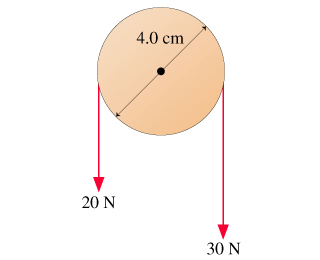# Problem: What is the net torque on the pulley about the axle?

###### FREE Expert Solution

Torque:

$\overline{){\mathbf{\tau }}{\mathbf{=}}{\mathbf{r}}{\mathbf{F}}}$

Counterclockwise torque is positive.

95% (124 ratings)###### Problem Details

What is the net torque on the pulley about the axle?